# Associativity facts for kids

Kids Encyclopedia Facts

Associative property is a property of mathematical operations (like addition and multiplication). It means that if you have more than one of the same associative operator (like +) in a row, the order of operations does not matter.

For example, if you have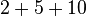$2+5+10\$, there are two plus signs (+) in a row. This means we can add it in either this order: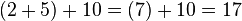$(2+5)+10=(7)+10=17\$

Or this order: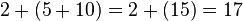$2+(5+10)=2+(15)=17\$

The answer comes out the same both ways because addition is associative. In other words, associativity means: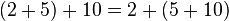$(2+5)+10=2+(5+10)\$

Not all operations are associative. Subtraction is not associative, which means: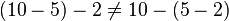$(10-5)-2\ne10-(5-2)$

This is true because: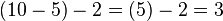$(10-5)-2=(5)-2=3\$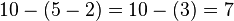$10-(5-2)=10-(3)=7\$

And: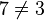$7\ne3$

Also, associativity is different from commutativity, which lets you move the numbers around.Associativity Facts for Kids. Kiddle Encyclopedia.